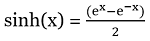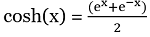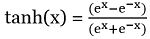# Hyperbolic Functions in valarray Class in C++

C++ valarray class Hyperbolic Functions: Learn about the popular hyperbolic functions with their usages and examples.
Submitted by Shivang Yadav, on May 20, 2022

The valarray class in C++ is a special container that is used for holding elements like an array and performing operations on them.

## Hyperbolic Functions

The hyperbolic functions take hyperbolic angles and are analogs of trigonometric values.

Basic hyperbolics functions,

1. Hyperbolic since denoted as sinh, formulated as2. Hyperbolic cosine denoted as cosh, formulated as3. Hyperbolic tangent denoted as tanh, formulated as### 1) sinh() function

The sinh() function in valarray class is used to return a valarray with values evaluated as sinh for each element of the original valarray.

Syntax:

```template< class T >
valarray<T> sinh( const valarray<T>& va );
```

Parameter(s): It accepts a single parameter which is the valarray whose elements as to be processed.

Return value: It returns a valarray consisting of values that are resultant of sinh.

Program to illustrate sinh() function on valarray

```#include <iostream>
#include <valarray>
using namespace std;

int main()
{
// Declaring valarray
valarray<double> myvalarr = { 1.6, -0.5, 0, 1, -1 };

// Printing the elements of valarray
cout << "The elements of orignal valarray are : ";
for (double& ele : myvalarr)
cout << ele << " ";

valarray<double> sinhvalarray = sinh(myvalarr);
cout << "\nThe elements of hyperbolic sine valarray are : ";
for (double& ele : sinhvalarray)
cout << ele << " ";

return 0;
}
```

Output:

```The elements of orignal valarray are : 1.6 -0.5 0 1 -1
The elements of hyperbolic sine valarray are : 2.37557 -0.521095 0 1.1752 -1.1752
```

### 2) cosh() function

The cosh() function in the valarray class is used to return a valarray with values evaluated as cosh for each element of the original valarray.

Syntax:

```template< class T >
valarray<T> cosh( const valarray<T>& va );
```

Parameter(s): It accepts a single parameter which is the valarray whose elements as to be processed.

Return value: It returns a valarray consisting of values that are resultant of cosh.

Program to illustrate the working of cosh() function

```#include <iostream>
#include <valarray>
using namespace std;

int main()
{
// Declaring valarray
valarray<double> myvalarr = { 1.6, -0.5, 0, 1, -1 };

// Printing the elements of valarray
cout << "The elements of orignal valarray are : ";
for (double& ele : myvalarr)
cout << ele << " ";

valarray<double> coshvalarray = cosh(myvalarr);
cout << "\nThe elements of hyperbolic cosine valarray are : ";
for (double& ele : coshvalarray)
cout << ele << " ";

return 0;
}
```

Output:

```The elements of orignal valarray are : 1.6 -0.5 0 1 -1
The elements of hyperbolic cosine valarray are : 2.57746 1.12763 1 1.54308 1.54308
```

### 3) tanh() function

The tanh() function in the valarray class is used to return a valarray with values evaluated as tanh for each element of the original valarray.

Syntax:

```template< class T >
valarray<T> cosh( const valarray<T>& va );
```

Parameter(s): It accepts a single parameter which is the valarray whose elements as to be processed.

Return value: It returns a valarray consisting of values that are resultant of tanh.

Program to show how the tanh() function is applied on valarray?

```#include <iostream>
#include <valarray>
using namespace std;

int main()
{
// Declaring valarray
valarray<double> myvalarr = { 1.6, -0.5, 0, 1, -1 };

// Printing the elements of valarray
cout << "The elements of orignal valarray are : ";
for (double& ele : myvalarr)
cout << ele << " ";

valarray<double> tanhvalarray = tanh(myvalarr);
cout << "\nThe elements of hyperbolic tangent valarray are : ";
for (double& ele : tanhvalarray)
cout << ele << " ";

return 0;
}
```

Output:

```The elements of orignal valarray are : 1.6 -0.5 0 1 -1
The elements of hyperbolic tangent valarray are : 0.921669 -0.462117 0 0.761594 -0.761594
```

Preparation

What's New

Top Interview Coding Problems/Challenges!